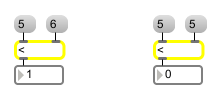# <

Is less than, comparison of two numbers

## Description

< takes any given number, compares it to another specified number, and outputs a 1 if the number is less than the comparison-number or 0 if it is greater or equal.

## Arguments

Name Type Opt Description
initial-comparison-value int or float opt Sets the initial value, to be compared with a number received in the left inlet. A float argument forces a float comparison.

## Messages

 bang In left inlet: Performs the comparison with the numbers currently stored. If there is no argument, < initially holds 0 for comparison. int input [int] In left inlet: If the number is less than the number in the right inlet, < outputs 1. Otherwise, < outputs 0. (inlet1) comparison-value [int] In right inlet: The number is stored to be compared with a number received in the left inlet. float input [float] Converted to int before comparison, unless < has a float argument. set set-input [int] The word set followed by a number will set the input value without causing output.

## Output

int: 1 if the number in the left inlet is less than the number in the right inlet. 0 if the number in the left inlet is greater than or equal to the number in the right inlet.

## ExamplesNumber on left is less than number on right... Number on left is not less than number on right